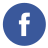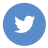### ParseFloat function in JavaScript

javascript

The parseFloat() function in JavaScript is used to parse a string argument and return a floating-point number. It takes one argument, which is the string to be parsed, and returns a floating-point number.

Here is the syntax for parseFloat():

``parseFloat(string)``

The string argument is the string that you want to parse as a floating-point number.

Here is an example of how to use parseFloat():

``````let numberString = "3.14159";
let number = parseFloat(numberString);

console.log(number); // 3.14159``````

In this example, the parseFloat() function is used to parse the string “3.14159” and return a floating-point number. The resulting number is stored in the number variable and then logged to the console.

It’s worth noting that if the first character in the string cannot be converted to a number, parseFloat() will return NaN (Not a Number). For example:

``````let numberString = "Hello, world!";
let number = parseFloat(numberString);

console.log(number); // NaN``````

In this example, the parseFloat() function is used to parse the string “Hello, world!”, which cannot be converted to a number. As a result, parseFloat() returns NaN.